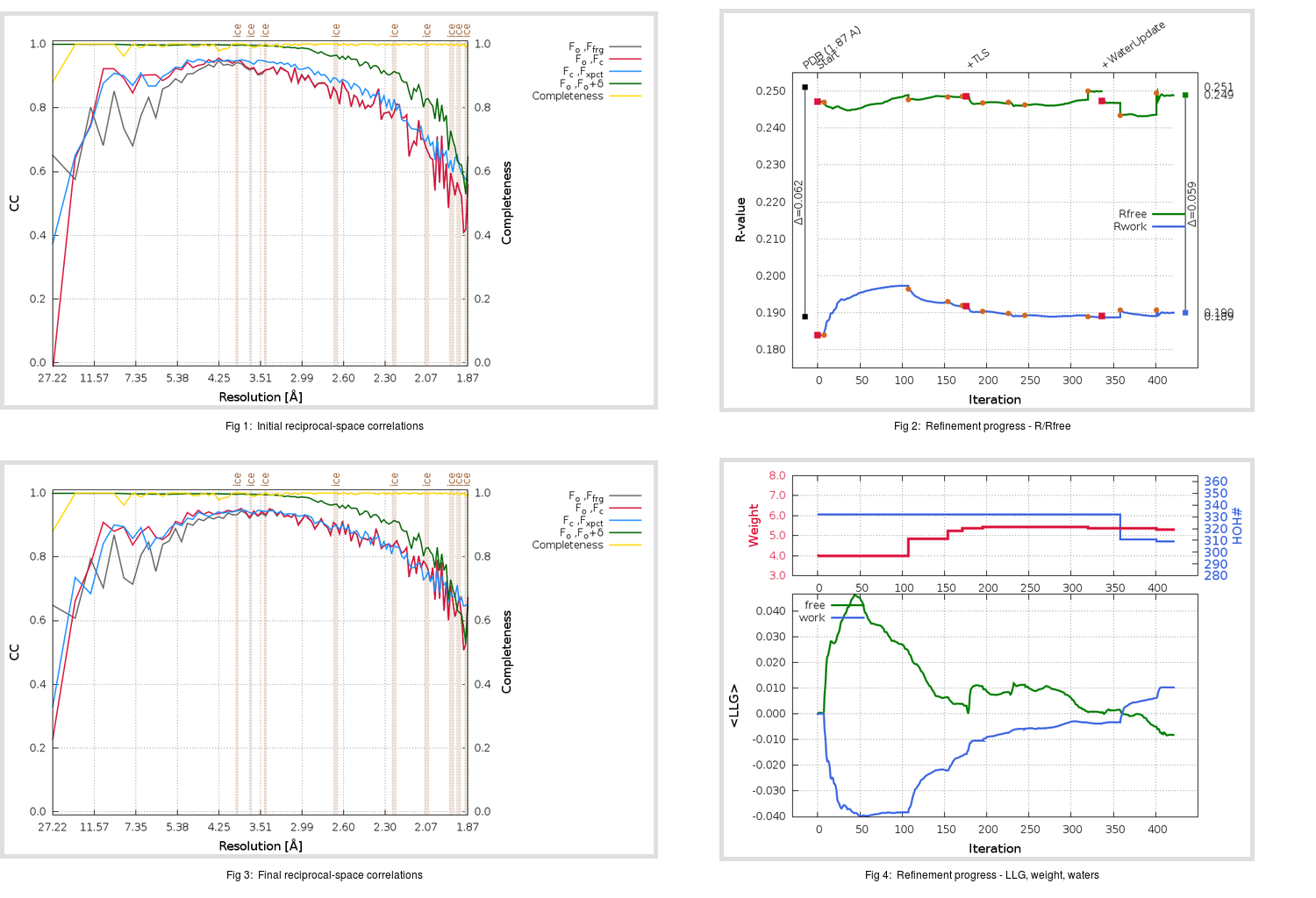Content:

## Deposited

` `
 Date deposited Date data collection Resolution R, Rfree 20200315 20200226 1.87 0.1860 0.2510

Molprobity (CCP4 7.0 version) summary:

```Ramachandran outliers =   0.33 %
favored =  96.36 %
Rotamer outliers      =   0.38 %
C-beta deviations     =     1
Clashscore            =   4.65
RMS(bonds)            =   0.0137
RMS(angles)           =   1.86
MolProbity score      =   1.48
Resolution            =   1.87
R-work                =   0.1860
R-free                =   0.2510
```

```Number of waters      =   332

<B> (all atoms) =   29.17 ( sd =    9.87 ) for       2731 non-hydrogen atoms
<B>   (protein) =   27.81 ( sd =    9.20 ) for       2370 non-hydrogen atoms
<B>     (water) =   38.06 ( sd =    9.61 ) for        332 non-hydrogen atoms
<B>    (others) =   38.90 ( sd =   12.08 ) for         29 non-hydrogen atoms

B min/max       (all non-hydrogen atoms) =   16.17 /   78.49
B min/max   (protein non-hydrogen atoms) =   16.17 /   75.41
B min/max     (water non-hydrogen atoms) =   16.42 /   78.49
B min/max     (other non-hydrogen atoms) =   28.57 /   50.75
```

## BUSTER (re-)refinement

` `

Molprobity (CCP4 7.0 version) summary:

```Ramachandran outliers =   0.33 %
favored =  98.01 %
Rotamer outliers      =   0.38 %
C-beta deviations     =     0
Clashscore            =   2.53
RMS(bonds)            =   0.0115
RMS(angles)           =   1.59
MolProbity score      =   1.04
Resolution            =   1.87
R-work                =   0.1900
R-free                =   0.2489
```

```Number of waters      =   309

<B> (all atoms) =   31.73 ( sd =    8.44 ) for       2708 non-hydrogen atoms
<B>   (protein) =   30.33 ( sd =    7.28 ) for       2370 non-hydrogen atoms
<B>     (water) =   41.71 ( sd =    9.36 ) for        309 non-hydrogen atoms
<B>    (others) =   39.63 ( sd =   12.74 ) for         29 non-hydrogen atoms

B min/max       (all non-hydrogen atoms) =   18.53 /   76.14
B min/max   (protein non-hydrogen atoms) =   19.65 /   66.91
B min/max     (water non-hydrogen atoms) =   18.53 /   76.14
B min/max     (other non-hydrogen atoms) =   34.24 /   50.82
```

Refinement progression:Results:

` `
 File Remark 5RE6_aB_refine.01_03_refine.pdb.gz exact refinement commands are in header 5RE6_aB_refine.01_03_refine.mtz.gz including original deposited data and several re-refinement map coefficients 5RE6_aB_refine.01_03_BUSTER_model.cif.gz including any non-standard compound restraints 5RE6_aB_refine.01_03_BUSTER_refln.cif.gz